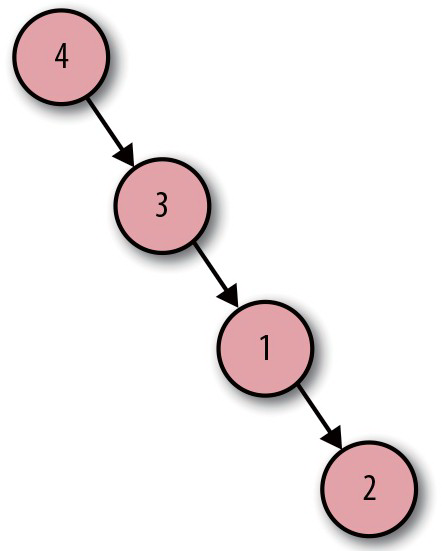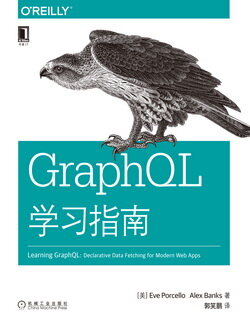# GraphQL 学习指南 (13)：图论 2.1(图论相关词汇)

《GraphQL 学习指南》还将向你介绍 Apollo Client，可用来将 GraphQL 连接到你的用户界面。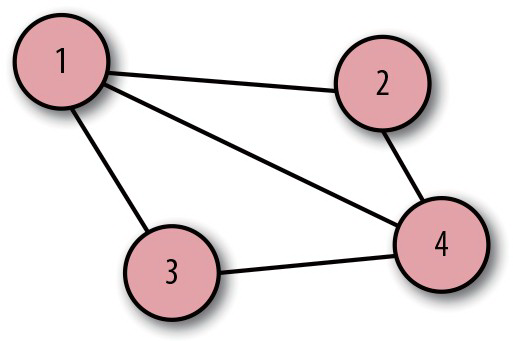1 更多关于节点和边的内容参见 Vaidehi Joshi 的博客：“A Gentle Introduction to Graph Theory”（ https://dev.to/vaidehijoshi/a-gentle-introduction-to-grph-theory ）。

    V = {1, 2, 3, 4}

$E$ 代表一组边。通常采用一对节点代表一条边。

    E = { {1, 2},          {1, 3},          {1, 4},          {2, 4},          {3, 4} }

    E = { {4, 3},          {4, 2},          {4, 1},          {3, 1},          {2, 1} }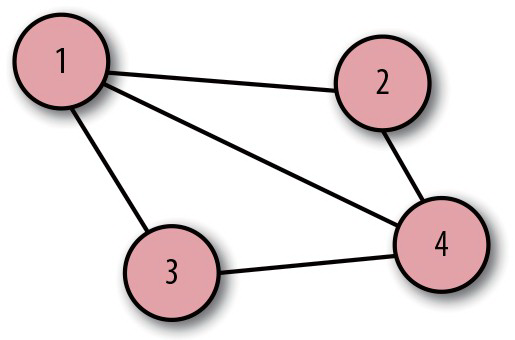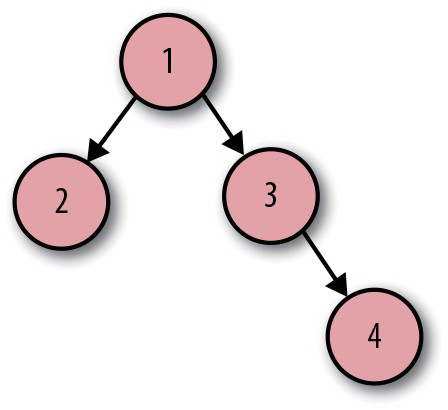V = {1, 2, 3, 4}    E = ( {1, 2},          {1, 3}          {3, 4} )

    G = ( {1, 2, 3, 4},          ({1, 2}, {1, 3}, {3, 4})  )

    G = ( {1, 2, 3, 4},          ( {4, 3}, {3, 1}, {1, 2} )  )